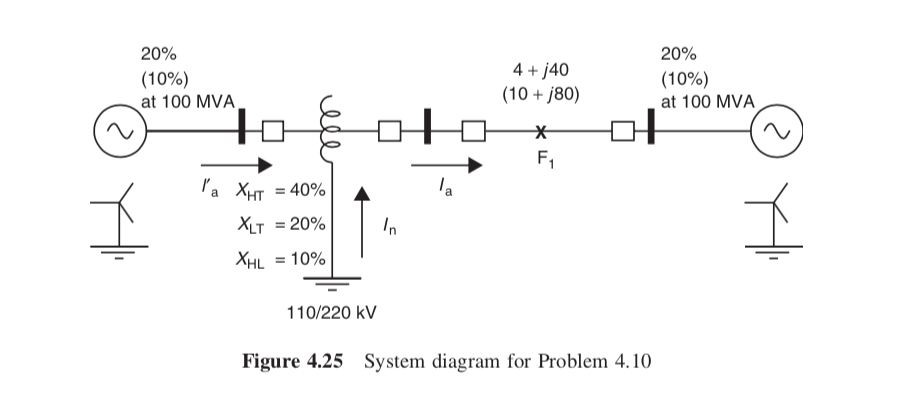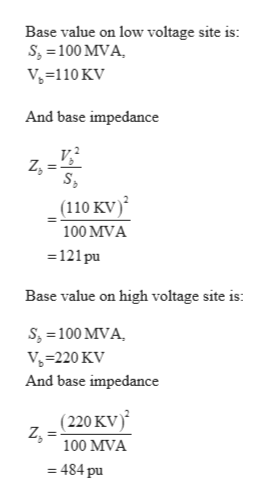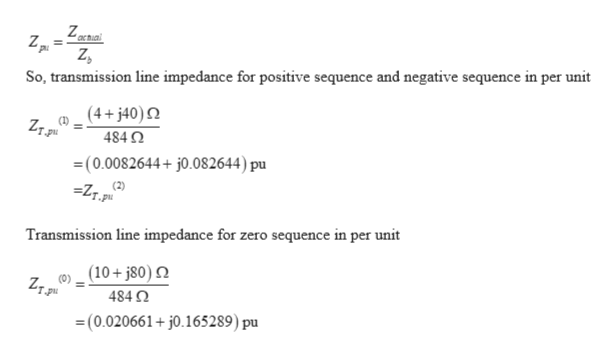# 20%20%4j40(10 j80)(10%at 100 MVA(10%at 100 MVAF1l'a XT =40%XLT 20%XHL 10%110/220 kVFigure 4.25 System diagram for Problem 4.10

Question
249 views

You are given that the system shown in Figure 4.25 has a 110/220 kV autotransformer. The positive- and zero-sequence impedances in ohms or percent are as shown in the figure, the zero-sequence impedances being in parentheses. Assume that the low- voltage system is solidly grounded. For a phase-a-to-ground fault at the midpoint of the transmission line, calculate the transformer current In in the neutral and the phase a currents Ia and I'a on the high and low sides of the transformer. If the source on the low-voltage side is to be grounded through a reactance, determine the value of the grounding reactance for which the transformer neutral current becomes zero. As the grounding reactance changes around this value, the direction of the neutral current will reverse, and will affect the polarizing capability of the neutral current for ground faults on the high side. Can faults on the low-voltage side ever cause the neutral current to reverse?help_outlineImage Transcriptionclose20% 20% 4j40 (10 j80) (10% at 100 MVA (10% at 100 MVA F1 l'a XT =40% XLT 20% XHL 10% 110/220 kV Figure 4.25 System diagram for Problem 4.10 fullscreen
check_circle

Step 1

Since there is single line to ground fault in phase a. So, fault current in phase a will be IF and fault current in other phases will be zero.

For a single line to ground fault, zero sequence fault current (Iaf(0)), positive sequence fault current (Iaf(1))  and negative sequence fault current (Iaf(2)) is calculated by Symmetrical component transformation matrix. This formula contains standard notation.

Step 2

Since positive sequence fault current is equal to the zero sequence fault current.

Calculate the zero sequence fault current (Iaf(0)) to get the current in neutral ground also current in high voltage and low voltage side of autotransformer.

So calculate the zero sequence fault current. For calculating the zero sequence fault current, draw the Thevenin’s equivalent circuit for positive sequence, negative sequence and zero sequence. Then calculate the equivalent Thevenin’s voltage and resistance for each sequence.

For solving the question further

Assumehelp_outlineImage TranscriptioncloseBase value on low voltage site is: s, 100 MVA V-110 KV And base impedance (110 KV) 100 MVA 121 pu Base value on high voltage site is: S 100 MVA V-220 KV And base impedance (220 KV) 100 MVA = 484 pu fullscreen
Step 3

Since transmission line is connected on high voltage site and impedance of transmission line is the actual value. So convert t...help_outlineImage TranscriptioncloseZ actial So, transmission line impedance for positive sequence and negative sequence in per unit (4+j40) 484 Q =(0.0082644+ j0.082644) pu (2) Transmission line impedance for zero sequence in per unit (10+j80) 484 Q (0.020661+j0.165289) pu fullscreen

### Want to see the full answer?

See Solution

#### Want to see this answer and more?

Solutions are written by subject experts who are available 24/7. Questions are typically answered within 1 hour.*

See Solution
*Response times may vary by subject and question.
Tagged in

### Electrical Engineering